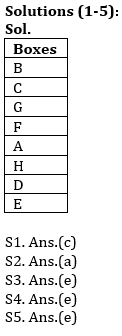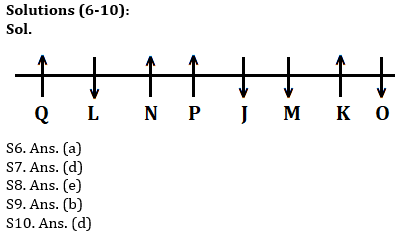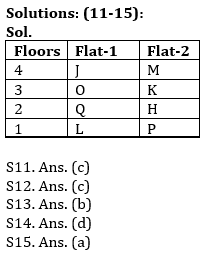Latest Banking jobs   »   Reasoning Ability Quiz For IBPS RRB...

# Reasoning Ability Quiz For IBPS RRB PO, Clerk Prelims 2021- 8th June

Directions (1-5): Read the following information carefully and answer the following questions.
Eight boxes A, B, C, D, E, F, G and H are place one above the other in any particular order. Box no. 1 is at the bottom and box no. 8 is at the top. Three boxes are placed between A and B. Box H is placed immediately below A. There are two boxes between H and G. There are as many boxes between C and D as between H and B. Box C is kept above D. Box E is kept immediately below box D. Three boxes are there between E and F.

Q1. How many boxes are there above box D?
(a) Four
(b) Three
(c) Six
(d) Two
(e) None of these

Q2. Which of the following box is kept at the top?
(a) B
(b) A
(c) D
(d) E
(e) None of these

Q3. Choose the odd one out?
(a) B-G
(b) G-A
(c) A-D
(d) C-F
(e) E-D

Q4. Which of the following boxes is kept between F and A?
(a) B
(b) G
(c) C
(d) H
(e) None

Q5. How many boxes are there between C and A?
(a) Less than Two
(b) Four
(c) Five
(d) Six
(e) None of these

Directions (6-10): Study the information carefully and answer the questions given below.
Eight persons J, K, L, M, N, O, P and Q are sitting in a linear row some of them facing towards the north and some of them facing towards south but not necessary in the same order. Not more than two consecutive persons face same direction.
N sits second to the right of J. Q sits second to the left of N. Two persons sit between Q and P. More than three persons sit between L and O. The persons sit on extreme ends face opposite direction to each other. M sits second to the right of P. N is not immediate neighbor of M, who sits third from one of the extreme ends of row. O is not immediate neighbor of N and M. O face south direction. J sits immediate right of M. L face opposite direction to Q.

Q6. Who among the following person sits second to right of O?
(a)M
(b)P
(c)J
(d)K
(e)None of these

Q7. How many person sits between N and K?
(a) One
(b) Two
(c) Four
(d) Three
(e)None of these

Q8. Who among the following person is immediate neighbor of P and J?
(a) L
(b) M
(c) K
(d) Q
(e) None of these

Q9. How many persons sits on the right of M?
(a) One
(b) More than Four
(c) Three
(d) Four
(e) None

Q10. Four of the following five are alike in certain way based from a group, find the one that does not belong to that group?
(a) Q
(b) N
(c) K
(d) J
(e) P

Directions (11-15): Study the following information carefully and answer the questions given below:
Eight persons are living in a building having four floors where ground floor is numbered as 1, the floor above it is numbered as 2 and so on till the topmost floor which is numbered as 4. Each floor is having two flats i.e., Flat-1 and flat-2. Flat-1 of floor-2 is exactly above the flat-1 of floor-1 and exactly below the flat-1 of floor-3 and so on. Similarly flat-2 of floor-2 is exactly above the flat-2 of floor-1 and exactly below flat-2 of floor-3 and so on. Flat-2 is in east of flat-1.
J lives to the west of M. There are two floor gap between L and J who does not live on bottom most floor. K lives on same numbered floor as O. O lives on a floor above Q’s floor who does not live in flat-2. There is one floor gap between M and H and both live on same numbered flat. One of the persons is P who does not live in flat-1. O lives on the same numbered flat as J.

Q11. Who among the following lives on flat-1 of 2nd floor?
(a)L
(b)M
(c)Q
(d)H
(e)None of these

Q12. Who among the following lives below P’s floor?
(a)H
(b)J
(c)No one
(d)O
(e)K

Q13. Four of the following five are alike in a certain way and hence form a group, which of the following does not belong to the group?
(a)L
(b)P
(c)Q
(d)O
(e)J

Q14. Which of the following statement/statements is/are true?
(a)J lives on the topmost floor
(b)K lives on a floor immediately above P’s floor
(c)H lives with Q on the same floor
(d)Both (a) and (c)
(e)Both (b) and (c)

Q15. Who among the following lives on the same numbered floor as P?
(a)L
(b)H
(c)M
(d)Q
(e)None of these

Practice More Questions of Reasoning for Competitive Exams:

###### Study Plan for IBPS RRB PO/Clerk Prelims 2021

Solutions#### Congratulations!